### 3.4 Filters

Filters are a method for calculating a variety of useful quantities from the field variables as the solution evolves in time, such as time-averaged fields and extracting the field variables at certain points inside the domain. Each filter is defined in a `FILTER` tag inside a `FILTERS` block which lies in the main `NEKTAR` tag. In this section we give an overview of the modules currently available and how to set up these filters in the session file.

Here is an example `FILTER`:

1<FILTER TYPE="FilterName">
2    <PARAM NAME="Param1"> Value1 </PARAM>
3    <PARAM NAME="Param2"> Value2 </PARAM>
4</FILTER>

A filter has a name – in this case, `FilterName` – together with parameters which are set to user-defined values. Each filter expects different parameter inputs, although where functionality is similar, the same parameter names are shared between filter types for consistency. Numerical filter parameters may be expressions and so may include session parameters defined in the `PARAMETERS` section.

In the following we document the filters implemented. Note that some filters are solver-specific and will therefore only work for a given subset of the available solvers.

#### 3.4.1 Time-averaged fields

This filter computes time-averaged fields for each variable defined in the session file. Time averages are computed by either taking a snapshot of the field every timestep, or alternatively at a user-defined number of timesteps N. An output is produced at the end of the simulation into `session_avg.fld`, or alternatively every M timesteps as defined by the user, into a sequence of files `session_*_avg.fld`, where `*` is replaced by a counter. This latter option can be useful to observe statistical convergence rates of the averaged variables.

The following parameters are supported:

 Option name Required Default Description `OutputFile` ✗ `session` Prefix of the output filename to which average fields are written. `SampleFrequency` ✗ 1 Number of timesteps at which the average is calculated, N. `OutputFrequency` ✗ `NumSteps` Number of timesteps after which output is written, M.

As an example, consider:

1<FILTER TYPE="AverageFields">
2    <PARAM NAME="OutputFile">MyAverageField</PARAM>
3    <PARAM NAME="OutputFrequency">100</PARAM>
4    <PARAM NAME="SampleFrequency"> 10 </PARAM>
5</FILTER>

This will create a file named `MyAverageField.fld` averaging the instantaneous fields every 10 time steps. The averaged field is however only output every 100 time steps.

#### 3.4.2 Moving average of fields

This filter computes the exponential moving average (in time) of fields for each variable defined in the session file. The moving average is defined as: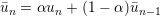with 0 < α < 1 and u1 = u1.

The same parameters of the time-average filter are supported, with the output file in the form `session_*_movAvg.fld`. In addition, either α or the time-constant τ must be defined. They are related by: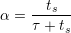where ts is the time interval between consecutive samples.

As an example, consider:

1<FILTER TYPE="MovingAverage">
2    <PARAM NAME="OutputFile">MyMovingAverage</PARAM>
3    <PARAM NAME="OutputFrequency">100</PARAM>
4    <PARAM NAME="SampleFrequency"> 10 </PARAM>
5    <PARAM NAME="tau"> 0.1 </PARAM>
6</FILTER>

This will create a file named `MyMovingAverage_movAvg.fld` with a moving average sampled every 10 time steps. The averaged field is however only output every 100 time steps.

#### 3.4.3 Reynolds stresses

Note: This filter is only supported for the incompressible Navier-Stokes solver.

This filter is an extended version of the time-average filter. It outputs not only the time-average of the fields, but also the Reynolds stresses. The same parameters supported in the time-average case can be used, for example:

1<FILTER TYPE="ReynoldsStresses">
2    <PARAM NAME="OutputFile">MyAverageField</PARAM>
3    <PARAM NAME="OutputFrequency">100</PARAM>
4    <PARAM NAME="SampleFrequency"> 10 </PARAM>
5</FILTER>

By default, this filter uses a simple average. Optionally, an exponential time average can be used, in which case the output contain the moving averages and the Reynolds stresses calculated based on them. For example:

1<FILTER TYPE="ReynoldsStresses">
2    <PARAM NAME="OutputFile">MyAverageField</PARAM>
3    <PARAM NAME="MovingAverage">true</PARAM>
4    <PARAM NAME="OutputFrequency">100</PARAM>
5    <PARAM NAME="SampleFrequency"> 10 </PARAM>
6    <PARAM NAME="alpha"> 0.01 </PARAM>
7</FILTER>

#### 3.4.4 Checkpoint fields

The checkpoint filter writes a checkpoint file, containing the instantaneous state of the solution fields at at given timestep. This can subsequently be used for restarting the simulation or examining time-dependent behaviour. This produces a sequence of files, by default named `session_*.chk`, where `*` is replaced by a counter. The initial condition is written to `session_0.chk`.

Note: This functionality is equivalent to setting the `IO_CheckSteps` parameter in the session file.

The following parameters are supported:

 Option name Required Default Description `OutputFile` ✗ `session` Prefix of the output filename to which the checkpoints are written. `OutputFrequency` ✓ - Number of timesteps after which output is written.

For example, to output the fields every 100 timesteps we can specify:

1<FILTER TYPE="Checkpoint">
2    <PARAM NAME="OutputFile">IntermediateFields</PARAM>
3    <PARAM NAME="OutputFrequency">100</PARAM>
4</FILTER>

#### 3.4.5 History points

The history points filter can be used to evaluate the value of the fields in specific points of the domain as the solution evolves in time. By default this produces a file called `session.his`. For each timestep, and then each history point, a line is output containing the current solution time, followed by the value of each of the field variables. Commented lines are created at the top of the file containing the location of the history points and the order of the variables.

The following parameters are supported:

 Option name Required Default Description `OutputFile` ✗ `session` Prefix of the output filename to which the checkpoints are written. `OutputFrequency` ✗ 1 Number of timesteps after which output is written. `OutputPlane` ✗ 0 If the simulation is homogeneous, the plane on which to evaluate the history point. (No Fourier interpolation is currently implemented.) `Points ` ✓ - A list of the history points. These should always be given in three dimensions.

For example, to output the value of the solution fields at three points (1,0.5,0), (2,0.5,0) and (3,0.5,0) into a file `TimeValues.his` every 10 timesteps, we use the syntax:

1<FILTER TYPE="HistoryPoints">
2    <PARAM NAME="OutputFile">TimeValues</PARAM>
3    <PARAM NAME="OutputFrequency">10</PARAM>
4    <PARAM NAME="Points">
5        1 0.5 0
6        2 0.5 0
7        3 0.5 0
8    </PARAM>
9</FILTER>

#### 3.4.6 ThresholdMax

The threshold value filter writes a field output containing a variable m, defined by the time at which the selected variable first exceeds a specified threshold value. The default name of the output file is the name of the session with the suffix `_max.fld`. Thresholding is applied based on the first variable listed in the session by default.

The following parameters are supported:

 Option name Required Default Description `OutputFile` ✗ session_max.fld Output filename to which the threshold times are written. `ThresholdVar` ✗ first variable name Specifies the variable on which the threshold will be applied. `ThresholdValue` ✓ - Specifies the threshold value. `InitialValue` ✓ - Specifies the initial time. `StartTime` ✗ 0.0 Specifies the time at which to start recording.

An example is given below:

1  <FILTER TYPE="ThresholdMax">
2      <PARAM NAME="OutputFile"> threshold_max.fld </PARAM>
3      <PARAM NAME="ThresholdVar"> u </PARAM>
4      <PARAM NAME="ThresholdValue"> 0.1 </PARAM>
5      <PARAM NAME="InitialValue">  0.4 </PARAM>
6  </FILTER>

which produces a field file `threshold_max.fld`.

#### 3.4.7 ThresholdMin value

Performs the same function as the `ThresholdMax` filter but records the time at which the threshold variable drops below a prescribed value.

#### 3.4.8 One-dimensional energy

This filter is designed to output the energy spectrum of one-dimensional elements. It transforms the solution field at each timestep into a orthogonal basis defined by the functions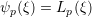where Lp is the p-th Legendre polynomial. This can be used to show the presence of, for example, oscillations in the underlying field due to numerical instability. The resulting output is written into a file called `session.eny` by default. The following parameters are supported:

 Option name Required Default Description `OutputFile` ✗ `session` Prefix of the output filename to which the energy spectrum is written. `OutputFrequency` ✗ 1 Number of timesteps after which output is written.

An example syntax is given below:

1<FILTER TYPE="Energy1D">
2    <PARAM NAME="OutputFile">EnergyFile</PARAM>
3    <PARAM NAME="OutputFrequency">10</PARAM>
4</FILTER>

#### 3.4.9 Modal energy

Note: This filter is only supported for the incompressible Navier-Stokes solver.

This filter calculates the time-evolution of the kinetic energy. In the case of a two- or three-dimensional simulation this is defined as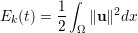However if the simulation is written as a one-dimensional homogeneous expansion so that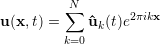then we instead calculate the energy spectrum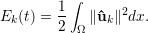Note that in this case, each component of ûk is a complex number and therefore N = `HomModesZ`∕2 lines are output for each timestep. This is a particularly useful tool in examining turbulent and transitional flows which use the homogeneous extension. In either case, the resulting output is written into a file called `session.mdl` by default.

The following parameters are supported:

 Option name Required Default Description `OutputFile` ✗ `session` Prefix of the output filename to which the energy spectrum is written. `OutputFrequency` ✗ 1 Number of timesteps after which output is written.

An example syntax is given below:

1<FILTER TYPE="ModalEnergy">
2    <PARAM NAME="OutputFile">EnergyFile</PARAM>
3    <PARAM NAME="OutputFrequency">10</PARAM>
4</FILTER>

#### 3.4.10 Aerodynamic forces

Note: This filter is only supported for the incompressible Navier-Stokes solver.

This filter evaluates the aerodynamic forces along a specific surface. The forces are projected along the Cartesian axes and the pressure and viscous contributions are computed in each direction.

The following parameters are supported:

 Option name Required Default Description `OutputFile` ✗ `session` Prefix of the output filename to which the forces are written. `Frequency` ✗ 1 Number of timesteps after which output is written. `Boundary` ✓ - Boundary surfaces on which the forces are to be evaluated.

An example is given below:

1  <FILTER TYPE="AeroForces">
2      <PARAM NAME="OutputFile">DragLift.frc</PARAM>
3      <PARAM NAME="OutputFrequency">10</PARAM>
4      <PARAM NAME="Boundary"> B[1,2] </PARAM>
5  </FILTER>

During the execution a file named `DragLift.frc` will be created and the value of the aerodynamic forces on boundaries 1 and 2, defined in the `GEOMETRY` section, will be output every 10 time steps.

#### 3.4.11 Kinetic energy and enstrophy

Note: This filter is only supported for the incompressible and compressible Navier-Stokes solvers in three dimensions.

The purpose of this filter is to calculate the kinetic energy and enstrophy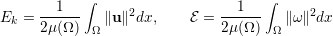where μ(Ω) is the volume of the domain Ω. This produces a file containing the time-evolution of the kinetic energy and enstrophy fields. By default this file is called `session.eny` where `session` is the session name.

The following parameters are supported:

 Option name Required Default Description `OutputFile` ✗ `session.eny` Output file name to which the energy and enstrophy are written. `OutputFrequency` ✓ - Number of timesteps at which output is written.

To enable the filter, add the following to the `FILTERS` tag:

1<FILTER TYPE="Energy">
2    <PARAM NAME="OutputFrequency"> 1 </PARAM>
3</FILTER>